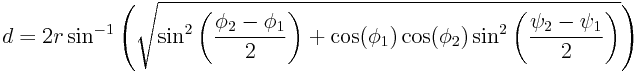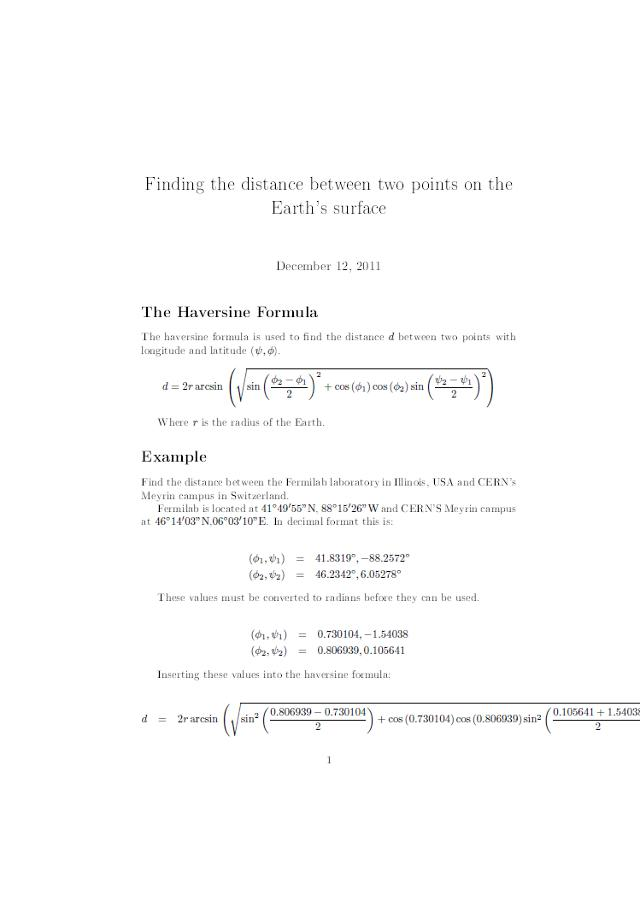# Haversine formula

The haversine formula is used to calculate the distance between two points on the Earth’s surface specified in longitude and latitude.d is the distance between two points with longitude and latitude (ψ,φ) and r is the radius of the Earth.

As an example I have calculated the distance between Fermilab in Illinois (41° 49′ 55″ N, 88° 15′ 26″ W) and CERN’s Meyrin campus in Switzerland (46° 14′ 3″ N, 6° 3′ 10″ E). There’s a little too much maths for this site to handle so I have included a .PDF file of the working below.The value calculated is 7084 km, which isn’t quite correct. This is because the formula assumes that the Earth is a perfect sphere when in fact it is an oblate spheroid. To compensate for this Vincenty’s Formulae must be used; these are much more complicated but give a more accurate value of 7103 km.

## One thought on “Haversine formula”

1. Anonymous says:

Good one. helped me so much.. Thanks